# VU Meter on Steroids: Arduino Nano and NeoPixel WS2812B

Responds to sound or can be set on automatic, all by IR. Or switch. WS2812B VU Bar on Uno or Nano. CHECK MY OTHER VU PROJECTS. And Builds.

IntermediateWork in progress25,380

## Things used in this project

### Hardware componentsArduino UNO & Genuino UNO
×1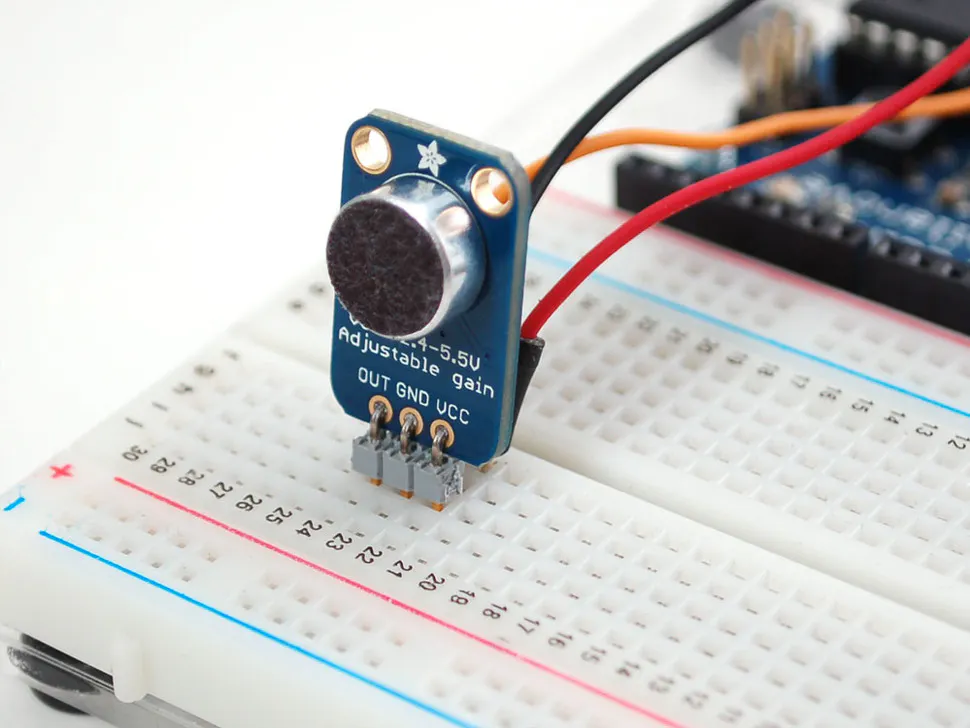Adafruit Microphone Amplifier Breakout
×1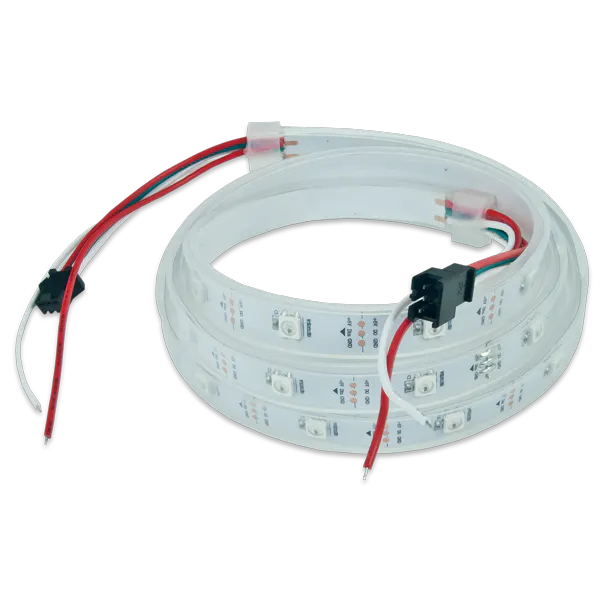Digilent WS2812 Addressable LED Strip
×1SparkFun Pushbutton switch 12mm
×1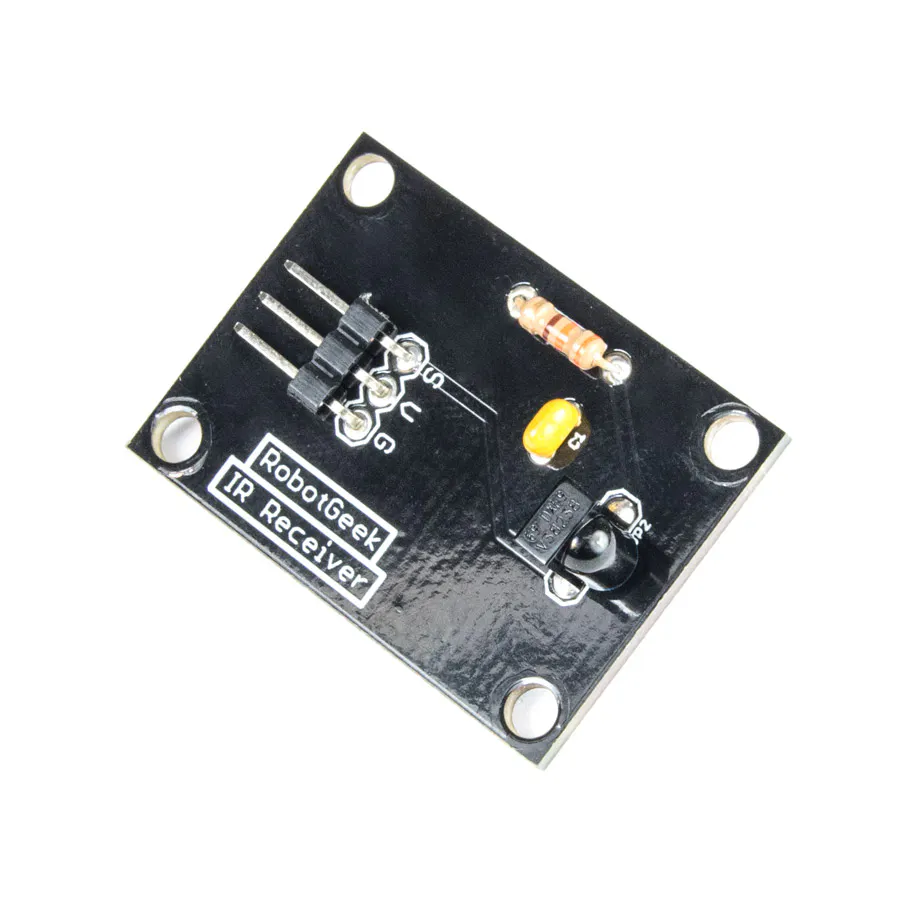×1Jumper wires (generic)
×1

### Hand tools and fabrication machinesSoldering iron (generic)

## Schematics

### Schematic picture and in code is the schematic for pinouts

in the code is everything explained on which pin you need to put the wires.
Pin 6 is for the WS2812B input ( + 5V 3 Amps EXTERNALY and common ground.
Pin 4 is the Switch or IR sensor ( and to ground of course )
Pin A5 is the Mic sensor.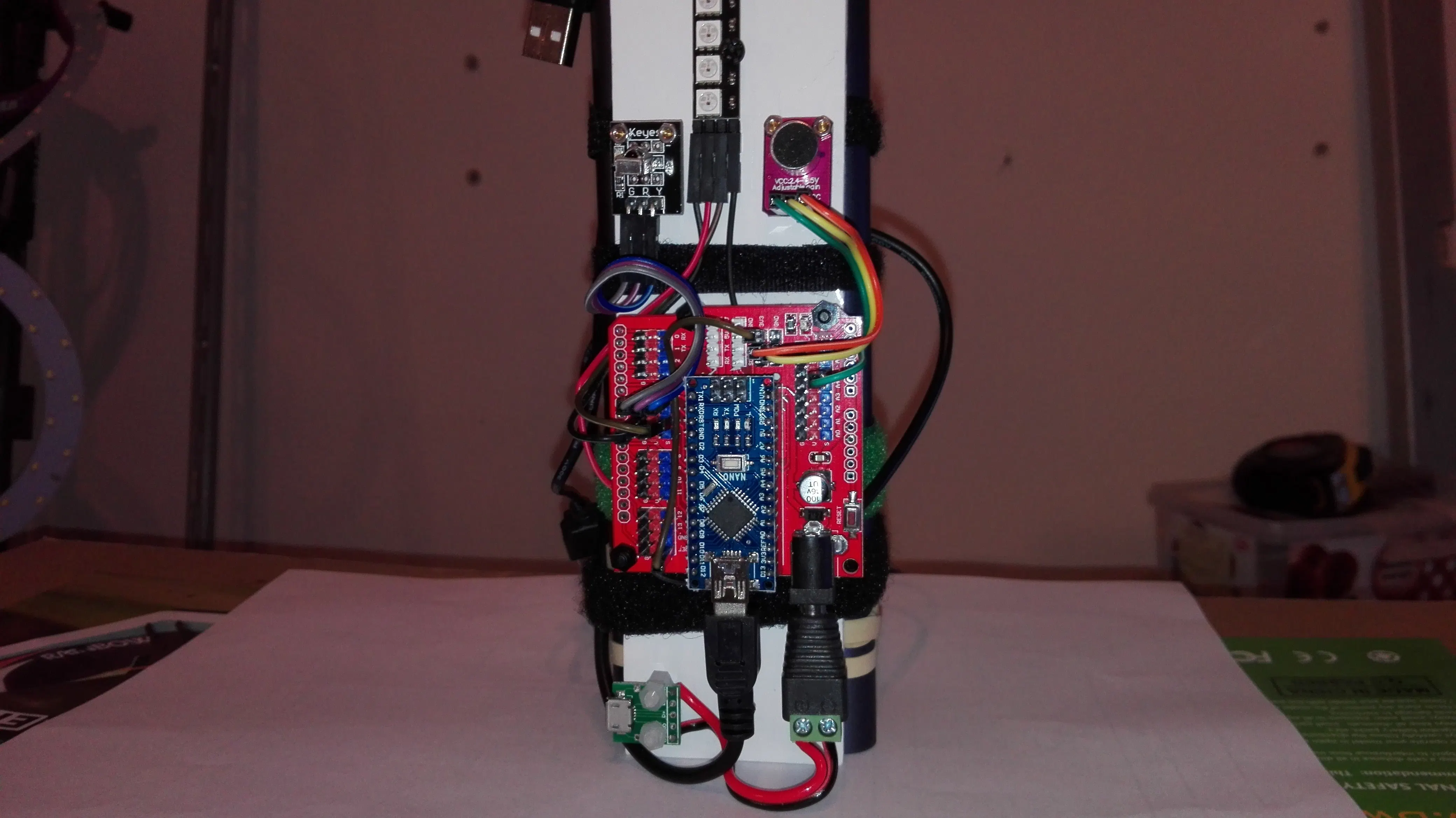### VU on Nano fritzing schematic

wire diagram on breadboard made BY ME.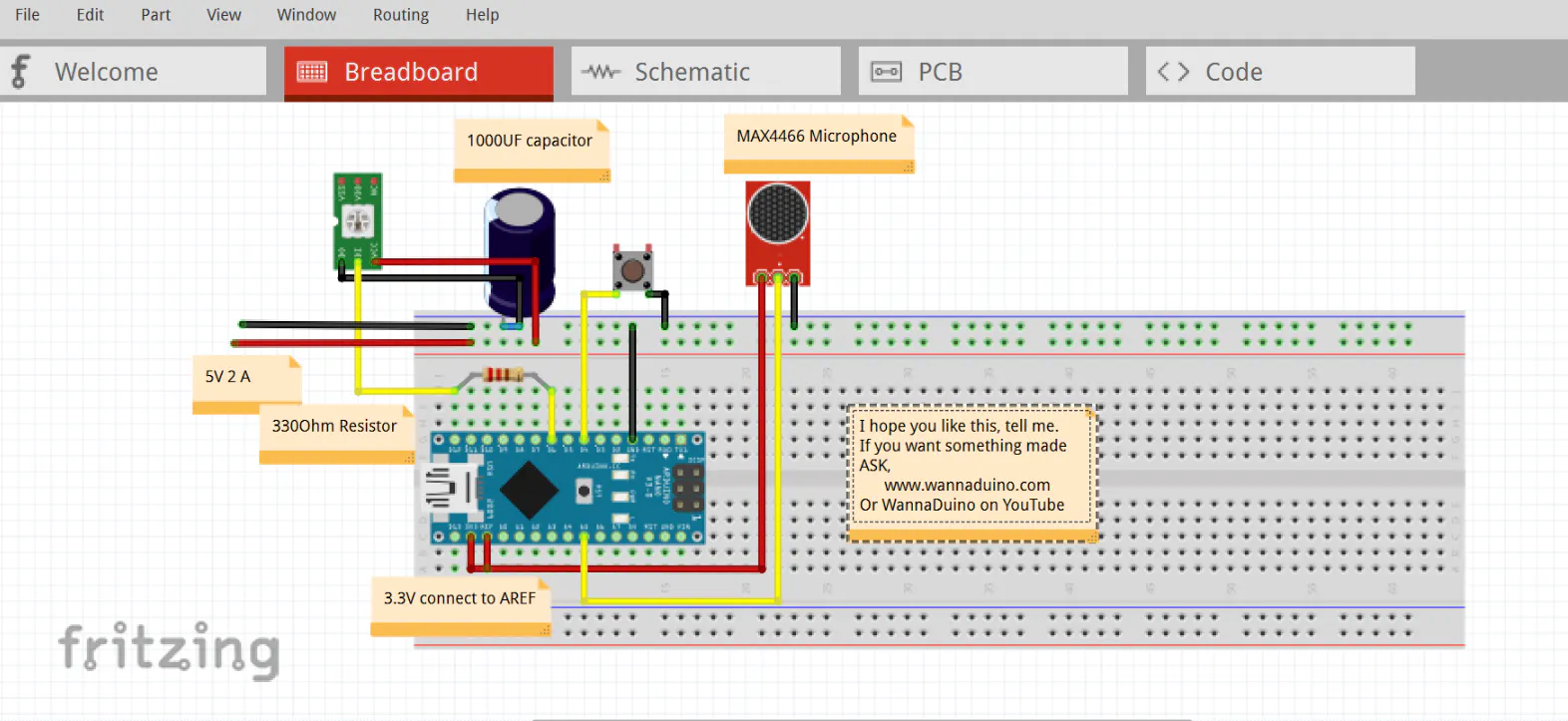### Vu on Nano FRITZING schematic

FRITZING file breadboard schematic wire diagram made by ME

## Code

### VU Meter on Nano or Uno

C/C++
https://youtu.be/9un-IkhvpLI
```#include <Adafruit_NeoPixel.h>
#include <FastLED.h>
#define PIN 6
#define N_PIXELS  60
#define BG 0
#define COLOR_ORDER GRB  // Try mixing up the letters (RGB, GBR, BRG, etc) for a whole new world of color combinations
#define BRIGHTNESS 255   // 0-255, higher number is brighter.
#define LED_TYPE WS2812B
#define MIC_PIN   A5  // Microphone is attached to this analog pin
#define DC_OFFSET  0  // DC offset in mic signal - if unusure, leave 0
#define NOISE     10  // Noise/hum/interference in mic signal
#define SAMPLES   60  // Length of buffer for dynamic level adjustment
#define TOP       (N_PIXELS + 2) // Allow dot to go slightly off scale
#define PEAK_FALL 40  // Rate of peak falling dot
#define N_PIXELS_HALF (N_PIXELS/2)
#define GRAVITY           -9.81              // Downward (negative) acceleration of gravity in m/s^2
#define h0                1                  // Starting height, in meters, of the ball (strip length)
#define NUM_BALLS         3                  // Number of bouncing balls you want (recommend < 7, but 20 is fun in its own way)
#define SPEED .20       // Amount to increment RGB color by each cycle

//config for balls
float h[NUM_BALLS] ;                         // An array of heights
float vImpact0 = sqrt( -2 * GRAVITY * h0 );  // Impact velocity of the ball when it hits the ground if "dropped" from the top of the strip
float vImpact[NUM_BALLS] ;                   // As time goes on the impact velocity will change, so make an array to store those values
float tCycle[NUM_BALLS] ;                    // The time since the last time the ball struck the ground
int   pos[NUM_BALLS] ;                       // The integer position of the dot on the strip (LED index)
long  tLast[NUM_BALLS] ;                     // The clock time of the last ground strike
float COR[NUM_BALLS] ;                       // Coefficient of Restitution (bounce damping)

float
greenOffset = 30,
blueOffset = 150;

byte
peak      = 0,      // Used for falling dot
dotCount  = 0,      // Frame counter for delaying dot-falling speed
volCount  = 0;      // Frame counter for storing past volume data
int
vol[SAMPLES],       // Collection of prior volume samples
lvl       = 10,      // Current "dampened" audio level
minLvlAvg = 0,      // For dynamic adjustment of graph low & high
maxLvlAvg = 512;

Adafruit_NeoPixel strip = Adafruit_NeoPixel(N_PIXELS, PIN, NEO_GRB + NEO_KHZ800);

// FOR SYLON ETC
uint8_t thisbeat =  23;
uint8_t thatbeat =  28;
uint8_t thisfade =   2;                                     // How quickly does it fade? Lower = slower fade rate.
uint8_t thissat = 255;                                     // The saturation, where 255 = brilliant colours.
uint8_t thisbri = 255;

//FOR JUGGLE
uint8_t numdots = 4;                                          // Number of dots in use.
uint8_t faderate = 2;                                         // How long should the trails be. Very low value = longer trails.
uint8_t hueinc = 16;                                          // Incremental change in hue between each dot.
uint8_t thishue = 0;                                          // Starting hue.
uint8_t curhue = 0;
uint8_t thisbright = 255;                                     // How bright should the LED/display be.
uint8_t basebeat = 5;
uint8_t max_bright = 255;

// Twinkle
float redStates[N_PIXELS];
float blueStates[N_PIXELS];
float greenStates[N_PIXELS];
float Fade = 0.96;

// Vu meter 4
const uint32_t Red = strip.Color(255, 0, 0);
const uint32_t Yellow = strip.Color(255, 255, 0);
const uint32_t Green = strip.Color(0, 255, 0);
const uint32_t Blue = strip.Color(0, 0, 255);
const uint32_t White = strip.Color(255, 255, 255);
const uint32_t Dark = strip.Color(0, 0, 0);
unsigned int sample;

CRGB leds[N_PIXELS];

int          myhue =   0;

// constants used here to set pin numbers:
const int buttonPin = 4;     // the number of the pushbutton pin

// Variables will change:

int buttonPushCounter = 0;   // counter for the number of button presses
int buttonState = 0;         // current state of the button
int lastButtonState = 0;

//Ripple variables
int color;
int center = 0;
int step = -1;
int maxSteps = 16;
float fadeRate = 0.80;
int diff;

//background color
uint32_t currentBg = random(256);
uint32_t nextBg = currentBg;

void setup() {
delay( 2000 ); // power-up safety delay
FastLED.addLeds<WS2812B, PIN, COLOR_ORDER>(leds, N_PIXELS).setCorrection( TypicalLEDStrip );
FastLED.setBrightness(  BRIGHTNESS );
analogReference(EXTERNAL);
memset(vol, 0, sizeof(vol));
LEDS.addLeds<LED_TYPE, PIN, COLOR_ORDER>(leds, N_PIXELS);
strip.begin();
strip.show(); // Initialize all pixels to 'off'

//initialize the serial port
Serial.begin(115200);
pinMode(buttonPin, INPUT);
//initialize the buttonPin as output
digitalWrite(buttonPin, HIGH);

for (int i = 0 ; i < NUM_BALLS ; i++) {    // Initialize variables
tLast[i] = millis();
h[i] = h0;
pos[i] = 0;                              // Balls start on the ground
vImpact[i] = vImpact0;                   // And "pop" up at vImpact0
tCycle[i] = 0;
COR[i] = 0.90 - float(i)/pow(NUM_BALLS,2);

}
}

void loop() {

//for mic
uint8_t  i;
uint16_t minLvl, maxLvl;
int      n, height;
// end mic

// read the pushbutton input pin:
// compare the buttonState to its previous state
if (buttonState != lastButtonState) {
// if the state has changed, increment the counter
if (buttonState == HIGH) {
// if the current state is HIGH then the button
// wend from off to on:
buttonPushCounter++;
Serial.println("on");
Serial.print("number of button pushes:  ");
Serial.println(buttonPushCounter);
if(buttonPushCounter==14) {
buttonPushCounter=1;}
}
else {
// if the current state is LOW then the button
// wend from on to off:
Serial.println("off");
}
}
// save the current state as the last state,
//for next time through the loop
lastButtonState = buttonState;

switch (buttonPushCounter){

case 1:
buttonPushCounter==1; {
vu(); // Red
break;}

case 2:
buttonPushCounter==2; {
vu2(); // Red
break;}

case 3:
buttonPushCounter==3; {
Vu3(); //
break;}

case 4:
buttonPushCounter==4; {
Vu4(); //
break;}

case 5:
buttonPushCounter==5; {
rainbow(150);
break;}

case 6:
buttonPushCounter==6; {
rainbow(20);
break;}

case 7:
buttonPushCounter==7; {
ripple();
break;}

case 8:
buttonPushCounter==8; {
ripple2();
break;}

case 9:
buttonPushCounter==9; {
Twinkle();
break;}

case 10:
buttonPushCounter==10; {
pattern2();
break;}

case 11:
buttonPushCounter==11; {
pattern3();
break;}

case 12:
buttonPushCounter==12; {
Balls(); //
break;}

case 13:
buttonPushCounter==13; {
colorWipe(strip.Color(0, 0, 0), 10); // A Black
break;}

}

}

void colorWipe(uint32_t c, uint8_t wait) {
for(uint16_t i=0; i<strip.numPixels(); i++) {
strip.setPixelColor(i, c);
strip.show();
if (digitalRead(buttonPin) != lastButtonState)  // <------------- add this
return;         // <------------ and this
delay(wait);
}
}
void Vu4() {
uint8_t  i;
uint16_t minLvl, maxLvl;
int      n, height;

n   = analogRead(MIC_PIN);                        // Raw reading from mic
n   = abs(n - 512 - DC_OFFSET); // Center on zero
n   = (n <= NOISE) ? 0 : (n - NOISE);             // Remove noise/hum
lvl = ((lvl * 7) + n) >> 3;    // "Dampened" reading (else looks twitchy)

// Calculate bar height based on dynamic min/max levels (fixed point):
height = TOP * (lvl - minLvlAvg) / (long)(maxLvlAvg - minLvlAvg);

if(height < 0L)       height = 0;      // Clip output
else if(height > TOP) height = TOP;
if(height > peak)     peak   = height; // Keep 'peak' dot at top
greenOffset += SPEED;
blueOffset += SPEED;
if (greenOffset >= 255) greenOffset = 0;
if (blueOffset >= 255) blueOffset = 0;

// Color pixels based on rainbow gradient
for(i=0; i<N_PIXELS_HALF; i++) {
if(i >= height) {
strip.setPixelColor(N_PIXELS_HALF-i-1,   0,   0, 0);
strip.setPixelColor(N_PIXELS_HALF+i,   0,   0, 0);
}
else {
uint32_t color = Wheel(map(i,0,N_PIXELS_HALF-1,(int)greenOffset, (int)blueOffset));
strip.setPixelColor(N_PIXELS_HALF-i-1,color);
strip.setPixelColor(N_PIXELS_HALF+i,color);
}

}

// Draw peak dot
if(peak > 0 && peak <= N_PIXELS_HALF-1) {
uint32_t color = Wheel(map(peak,0,N_PIXELS_HALF-1,30,150));
strip.setPixelColor(N_PIXELS_HALF-peak-1,color);
strip.setPixelColor(N_PIXELS_HALF+peak,color);
}

strip.show(); // Update strip

// Every few frames, make the peak pixel drop by 1:

if(++dotCount >= PEAK_FALL) { //fall rate

if(peak > 0) peak--;
dotCount = 0;
}

vol[volCount] = n;                      // Save sample for dynamic leveling
if(++volCount >= SAMPLES) volCount = 0; // Advance/rollover sample counter

// Get volume range of prior frames
minLvl = maxLvl = vol;
for(i=1; i<SAMPLES; i++) {
if(vol[i] < minLvl)      minLvl = vol[i];
else if(vol[i] > maxLvl) maxLvl = vol[i];
}
// minLvl and maxLvl indicate the volume range over prior frames, used
// for vertically scaling the output graph (so it looks interesting
// regardless of volume level).  If they're too close together though
// (e.g. at very low volume levels) the graph becomes super coarse
// and 'jumpy'...so keep some minimum distance between them (this
// also lets the graph go to zero when no sound is playing):
if((maxLvl - minLvl) < TOP) maxLvl = minLvl + TOP;
minLvlAvg = (minLvlAvg * 63 + minLvl) >> 6; // Dampen min/max levels
maxLvlAvg = (maxLvlAvg * 63 + maxLvl) >> 6; // (fake rolling average)

}

void Vu3() {
uint8_t i;
uint16_t minLvl, maxLvl;
int n, height;

n = analogRead(MIC_PIN);             // Raw reading from mic
n = abs(n - 512 - DC_OFFSET);        // Center on zero
n = (n <= NOISE) ? 0 : (n - NOISE);  // Remove noise/hum
lvl = ((lvl * 7) + n) >> 3;    // "Dampened" reading (else looks twitchy)

// Calculate bar height based on dynamic min/max levels (fixed point):
height = TOP * (lvl - minLvlAvg) / (long)(maxLvlAvg - minLvlAvg);

if (height < 0L)       height = 0;      // Clip output
else if (height > TOP) height = TOP;
if (height > peak)     peak   = height; // Keep 'peak' dot at top

greenOffset += SPEED;
blueOffset += SPEED;
if (greenOffset >= 255) greenOffset = 0;
if (blueOffset >= 255) blueOffset = 0;

// Color pixels based on rainbow gradient
for (i = 0; i < N_PIXELS; i++) {
if (i >= height) {
strip.setPixelColor(i, 0, 0, 0);
} else {
strip.setPixelColor(i, Wheel(
map(i, 0, strip.numPixels() - 1, (int)greenOffset, (int)blueOffset)
));
}
}
// Draw peak dot
if(peak > 0 && peak <= N_PIXELS-1) strip.setPixelColor(peak,Wheel(map(peak,0,strip.numPixels()-1,30,150)));

strip.show(); // Update strip

// Every few frames, make the peak pixel drop by 1:

if(++dotCount >= PEAK_FALL) { //fall rate

if(peak > 0) peak--;
dotCount = 0;
}
strip.show();  // Update strip

vol[volCount] = n;
if (++volCount >= SAMPLES) {
volCount = 0;
}

// Get volume range of prior frames
minLvl = maxLvl = vol;
for (i = 1; i < SAMPLES; i++) {
if (vol[i] < minLvl) {
minLvl = vol[i];
} else if (vol[i] > maxLvl) {
maxLvl = vol[i];
}
}

// minLvl and maxLvl indicate the volume range over prior frames, used
// for vertically scaling the output graph (so it looks interesting
// regardless of volume level).  If they're too close together though
// (e.g. at very low volume levels) the graph becomes super coarse
// and 'jumpy'...so keep some minimum distance between them (this
// also lets the graph go to zero when no sound is playing):
if ((maxLvl - minLvl) < TOP) {
maxLvl = minLvl + TOP;
}
minLvlAvg = (minLvlAvg * 63 + minLvl) >> 6; // Dampen min/max levels
maxLvlAvg = (maxLvlAvg * 63 + maxLvl) >> 6; // (fake rolling average)
}

void Balls() {
for (int i = 0 ; i < NUM_BALLS ; i++) {
tCycle[i] =  millis() - tLast[i] ;     // Calculate the time since the last time the ball was on the ground

// A little kinematics equation calculates positon as a function of time, acceleration (gravity) and intial velocity
h[i] = 0.5 * GRAVITY * pow( tCycle[i]/1000 , 2.0 ) + vImpact[i] * tCycle[i]/1000;

if ( h[i] < 0 ) {
h[i] = 0;                            // If the ball crossed the threshold of the "ground," put it back on the ground
vImpact[i] = COR[i] * vImpact[i] ;   // and recalculate its new upward velocity as it's old velocity * COR
tLast[i] = millis();

if ( vImpact[i] < 0.01 ) vImpact[i] = vImpact0;  // If the ball is barely moving, "pop" it back up at vImpact0
}
pos[i] = round( h[i] * (N_PIXELS - 1) / h0);       // Map "h" to a "pos" integer index position on the LED strip
}

//Choose color of LEDs, then the "pos" LED on
for (int i = 0 ; i < NUM_BALLS ; i++) leds[pos[i]] = CHSV( uint8_t (i * 40) , 255, 255);
FastLED.show();
//Then off for the next loop around
for (int i = 0 ; i < NUM_BALLS ; i++) {
leds[pos[i]] = CRGB::Black;
}
}

// Slightly different, this makes the rainbow equally distributed throughout
void rainbowCycle(uint8_t wait) {
uint16_t i, j;

for(j=0; j<256*5; j++) { // 5 cycles of all colors on wheel
for(i=0; i< strip.numPixels(); i++) {
strip.setPixelColor(i, Wheel(((i * 256 / strip.numPixels()) + j) & 255));
}
strip.show();
if (digitalRead(buttonPin) != lastButtonState)  // <------------- add this
return;         // <------------ and this
delay(wait);
}
}
// HERE

void vu() {

uint8_t  i;
uint16_t minLvl, maxLvl;
int      n, height;

n   = analogRead(MIC_PIN);                        // Raw reading from mic
n   = abs(n - 512 - DC_OFFSET); // Center on zero
n   = (n <= NOISE) ? 0 : (n - NOISE);             // Remove noise/hum
lvl = ((lvl * 7) + n) >> 3;    // "Dampened" reading (else looks twitchy)

// Calculate bar height based on dynamic min/max levels (fixed point):
height = TOP * (lvl - minLvlAvg) / (long)(maxLvlAvg - minLvlAvg);

if(height < 0L)       height = 0;      // Clip output
else if(height > TOP) height = TOP;
if(height > peak)     peak   = height; // Keep 'peak' dot at top

// Color pixels based on rainbow gradient
for(i=0; i<N_PIXELS; i++) {
if(i >= height)               strip.setPixelColor(i,   0,   0, 0);
else strip.setPixelColor(i,Wheel(map(i,0,strip.numPixels()-1,30,150)));

}

// Draw peak dot
if(peak > 0 && peak <= N_PIXELS-1) strip.setPixelColor(peak,Wheel(map(peak,0,strip.numPixels()-1,30,150)));

strip.show(); // Update strip

// Every few frames, make the peak pixel drop by 1:

if(++dotCount >= PEAK_FALL) { //fall rate

if(peak > 0) peak--;
dotCount = 0;
}

vol[volCount] = n;                      // Save sample for dynamic leveling
if(++volCount >= SAMPLES) volCount = 0; // Advance/rollover sample counter

// Get volume range of prior frames
minLvl = maxLvl = vol;
for(i=1; i<SAMPLES; i++) {
if(vol[i] < minLvl)      minLvl = vol[i];
else if(vol[i] > maxLvl) maxLvl = vol[i];
}
// minLvl and maxLvl indicate the volume range over prior frames, used
// for vertically scaling the output graph (so it looks interesting
// regardless of volume level).  If they're too close together though
// (e.g. at very low volume levels) the graph becomes super coarse
// and 'jumpy'...so keep some minimum distance between them (this
// also lets the graph go to zero when no sound is playing):
if((maxLvl - minLvl) < TOP) maxLvl = minLvl + TOP;
minLvlAvg = (minLvlAvg * 63 + minLvl) >> 6; // Dampen min/max levels
maxLvlAvg = (maxLvlAvg * 63 + maxLvl) >> 6; // (fake rolling average)

}

// Input a value 0 to 255 to get a color value.
// The colors are a transition r - g - b - back to r.
uint32_t Wheel(byte WheelPos) {
if(WheelPos < 85) {
return strip.Color(WheelPos * 3, 255 - WheelPos * 3, 0);
} else if(WheelPos < 170) {
WheelPos -= 85;
return strip.Color(255 - WheelPos * 3, 0, WheelPos * 3);
} else {
WheelPos -= 170;
return strip.Color(0, WheelPos * 3, 255 - WheelPos * 3);
}
}

void vu2() {

uint8_t  i;
uint16_t minLvl, maxLvl;
int      n, height;

n   = analogRead(MIC_PIN);                        // Raw reading from mic
n   = abs(n - 512 - DC_OFFSET); // Center on zero
n   = (n <= NOISE) ? 0 : (n - NOISE);             // Remove noise/hum
lvl = ((lvl * 7) + n) >> 3;    // "Dampened" reading (else looks twitchy)

// Calculate bar height based on dynamic min/max levels (fixed point):
height = TOP * (lvl - minLvlAvg) / (long)(maxLvlAvg - minLvlAvg);

if(height < 0L)       height = 0;      // Clip output
else if(height > TOP) height = TOP;
if(height > peak)     peak   = height; // Keep 'peak' dot at top

// Color pixels based on rainbow gradient
for(i=0; i<N_PIXELS_HALF; i++) {
if(i >= height) {
strip.setPixelColor(N_PIXELS_HALF-i-1,   0,   0, 0);
strip.setPixelColor(N_PIXELS_HALF+i,   0,   0, 0);
}
else {
uint32_t color = Wheel(map(i,0,N_PIXELS_HALF-1,30,150));
strip.setPixelColor(N_PIXELS_HALF-i-1,color);
strip.setPixelColor(N_PIXELS_HALF+i,color);
}

}

// Draw peak dot
if(peak > 0 && peak <= N_PIXELS_HALF-1) {
uint32_t color = Wheel(map(peak,0,N_PIXELS_HALF-1,30,150));
strip.setPixelColor(N_PIXELS_HALF-peak-1,color);
strip.setPixelColor(N_PIXELS_HALF+peak,color);
}

strip.show(); // Update strip

// Every few frames, make the peak pixel drop by 1:

if(++dotCount >= PEAK_FALL) { //fall rate

if(peak > 0) peak--;
dotCount = 0;
}

vol[volCount] = n;                      // Save sample for dynamic leveling
if(++volCount >= SAMPLES) volCount = 0; // Advance/rollover sample counter

// Get volume range of prior frames
minLvl = maxLvl = vol;
for(i=1; i<SAMPLES; i++) {
if(vol[i] < minLvl)      minLvl = vol[i];
else if(vol[i] > maxLvl) maxLvl = vol[i];
}
// minLvl and maxLvl indicate the volume range over prior frames, used
// for vertically scaling the output graph (so it looks interesting
// regardless of volume level).  If they're too close together though
// (e.g. at very low volume levels) the graph becomes super coarse
// and 'jumpy'...so keep some minimum distance between them (this
// also lets the graph go to zero when no sound is playing):
if((maxLvl - minLvl) < TOP) maxLvl = minLvl + TOP;
minLvlAvg = (minLvlAvg * 63 + minLvl) >> 6; // Dampen min/max levels
maxLvlAvg = (maxLvlAvg * 63 + maxLvl) >> 6; // (fake rolling average)

}

//here................

void ripple() {

if (currentBg == nextBg) {
nextBg = random(256);
}
else if (nextBg > currentBg) {
currentBg++;
} else {
currentBg--;
}
for(uint16_t l = 0; l < N_PIXELS; l++) {
leds[l] = CHSV(currentBg, 255, 50);         // strip.setPixelColor(l, Wheel(currentBg, 0.1));
}

if (step == -1) {
center = random(N_PIXELS);
color = random(256);
step = 0;
}

if (step == 0) {
leds[center] = CHSV(color, 255, 255);         // strip.setPixelColor(center, Wheel(color, 1));
step ++;
}
else {
if (step < maxSteps) {

leds[wrap(center + step)] = CHSV(color, 255, pow(fadeRate, step)*255);       //   strip.setPixelColor(wrap(center + step), Wheel(color, pow(fadeRate, step)));
leds[wrap(center - step)] = CHSV(color, 255, pow(fadeRate, step)*255);       //   strip.setPixelColor(wrap(center - step), Wheel(color, pow(fadeRate, step)));
if (step > 3) {
leds[wrap(center + step - 3)] = CHSV(color, 255, pow(fadeRate, step - 2)*255);     //   strip.setPixelColor(wrap(center + step - 3), Wheel(color, pow(fadeRate, step - 2)));
leds[wrap(center - step + 3)] = CHSV(color, 255, pow(fadeRate, step - 2)*255);     //   strip.setPixelColor(wrap(center - step + 3), Wheel(color, pow(fadeRate, step - 2)));
}
step ++;
}
else {
step = -1;
}
}

LEDS.show();
delay(50);
}

int wrap(int step) {
if(step < 0) return N_PIXELS + step;
if(step > N_PIXELS - 1) return step - N_PIXELS;
return step;
}

void one_color_allHSV(int ahue, int abright) {                // SET ALL LEDS TO ONE COLOR (HSV)
for (int i = 0 ; i < N_PIXELS; i++ ) {
leds[i] = CHSV(ahue, 255, abright);
}
}

void ripple2() {
if (BG){
if (currentBg == nextBg) {
nextBg = random(256);
}
else if (nextBg > currentBg) {
currentBg++;
} else {
currentBg--;
}
for(uint16_t l = 0; l < N_PIXELS; l++) {
strip.setPixelColor(l, Wheel(currentBg, 0.1));
}
} else {
for(uint16_t l = 0; l < N_PIXELS; l++) {
strip.setPixelColor(l, 0, 0, 0);
}
}

if (step == -1) {
center = random(N_PIXELS);
color = random(256);
step = 0;
}

if (step == 0) {
strip.setPixelColor(center, Wheel(color, 1));
step ++;
}
else {
if (step < maxSteps) {
strip.setPixelColor(wrap(center + step), Wheel(color, pow(fadeRate, step)));
strip.setPixelColor(wrap(center - step), Wheel(color, pow(fadeRate, step)));
if (step > 3) {
strip.setPixelColor(wrap(center + step - 3), Wheel(color, pow(fadeRate, step - 2)));
strip.setPixelColor(wrap(center - step + 3), Wheel(color, pow(fadeRate, step - 2)));
}
step ++;
}
else {
step = -1;
}
}

strip.show();
delay(50);
}
//int wrap(int step) {
//  if(step < 0) return Pixels + step;
//  if(step > Pixels - 1) return step - Pixels;
//  return step;
//}

// Input a value 0 to 255 to get a color value.
// The colours are a transition r - g - b - back to r.
uint32_t Wheel(byte WheelPos, float opacity) {

if(WheelPos < 85) {
return strip.Color((WheelPos * 3) * opacity, (255 - WheelPos * 3) * opacity, 0);
}
else if(WheelPos < 170) {
WheelPos -= 85;
return strip.Color((255 - WheelPos * 3) * opacity, 0, (WheelPos * 3) * opacity);
}
else {
WheelPos -= 170;
return strip.Color(0, (WheelPos * 3) * opacity, (255 - WheelPos * 3) * opacity);
}
}

void pattern2() {

sinelon();                                                  // Call our sequence.
show_at_max_brightness_for_power();                         // Power managed display of LED's.
} // loop()

void sinelon() {
// a colored dot sweeping back and forth, with fading trails
int pos1 = beatsin16(thisbeat,0,N_PIXELS);
int pos2 = beatsin16(thatbeat,0,N_PIXELS);
leds[(pos1+pos2)/2] += CHSV( myhue++/64, thissat, thisbri);
}
// Pattern 3 - JUGGLE
void pattern3() {
ChangeMe();
juggle();
show_at_max_brightness_for_power();                         // Power managed display of LED's.
} // loop()

void juggle() {                                               // Several colored dots, weaving in and out of sync with each other
curhue = thishue;                                          // Reset the hue values.
for( int i = 0; i < numdots; i++) {
leds[beatsin16(basebeat+i+numdots,0,N_PIXELS)] += CHSV(curhue, thissat, thisbright);   //beat16 is a FastLED 3.1 function
curhue += hueinc;
}
} // juggle()

void ChangeMe() {                                             // A time (rather than loop) based demo sequencer. This gives us full control over the length of each sequence.
uint8_t secondHand = (millis() / 1000) % 30;                // IMPORTANT!!! Change '30' to a different value to change duration of the loop.
static uint8_t lastSecond = 99;                             // Static variable, means it's only defined once. This is our 'debounce' variable.
if (lastSecond != secondHand) {                             // Debounce to make sure we're not repeating an assignment.
lastSecond = secondHand;
if (secondHand ==  0)  {numdots=1; faderate=2;}  // You can change values here, one at a time , or altogether.
if (secondHand == 10)  {numdots=4; thishue=128; faderate=8;}
if (secondHand == 20)  {hueinc=48; thishue=random8();}                               // Only gets called once, and not continuously for the next several seconds. Therefore, no rainbows.
}
} // ChangeMe()

void Twinkle () {
if (random(25) == 1) {
uint16_t i = random(N_PIXELS);
if (redStates[i] < 1 && greenStates[i] < 1 && blueStates[i] < 1) {
redStates[i] = random(256);
greenStates[i] = random(256);
blueStates[i] = random(256);
}
}

for(uint16_t l = 0; l < N_PIXELS; l++) {
if (redStates[l] > 1 || greenStates[l] > 1 || blueStates[l] > 1) {
strip.setPixelColor(l, redStates[l], greenStates[l], blueStates[l]);

if (redStates[l] > 1) {
redStates[l] = redStates[l] * Fade;
} else {
redStates[l] = 0;
}

if (greenStates[l] > 1) {
greenStates[l] = greenStates[l] * Fade;
} else {
greenStates[l] = 0;
}

if (blueStates[l] > 1) {
blueStates[l] = blueStates[l] * Fade;
} else {
blueStates[l] = 0;
}

} else {
strip.setPixelColor(l, 0, 0, 0);
}
}
strip.show();
delay(10);

}

// TOO HERE

void rainbow(uint8_t wait) {
uint16_t i, j;

for(j=0; j<256; j++) {
for(i=0; i<strip.numPixels(); i++) {
strip.setPixelColor(i, Wheel((i+j) & 255));
}
strip.show();
// check if a button pressed
if (digitalRead(buttonPin) != lastButtonState)  // <------------- add this
return;         // <------------ and this
delay(wait);
}
}
```